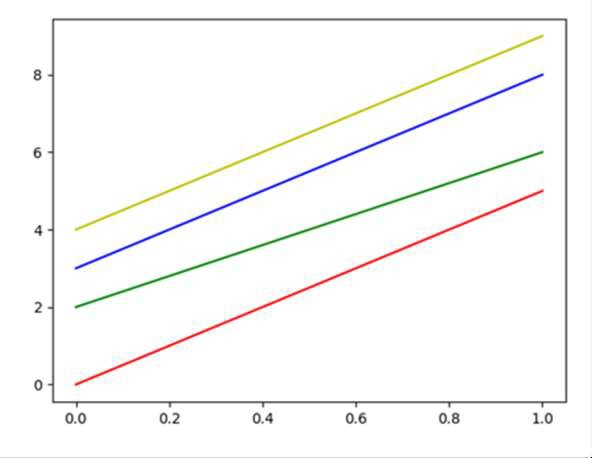# How can I cycle through line styles in Matplotlib?

To plot multiple lines in a diagram, we can use the cycler that could help to set a new color from the given list of colors. (Here, ‘r’ => ‘red’, ‘g’ => ‘green’, ‘y’ => ‘yellow’, ‘b’ => ‘blue’).

## Steps

• Use a cycler to set the color for the group of lines. The color list consists of ‘r’ for red, ‘g’ for green, ‘b’ for blue, and ‘y’ for yellow.

• The cycler class helps to create a new Cycler object from a single positional argument, a pair of positional arguments, or the combination of keyword arguments.

• Plot the number of lines with different colors.

• Use plt.show() to show the figure.

## Example

import matplotlib.pyplot as plt
from cycler import cycler

plt.rc('axes', prop_cycle=(cycler('color', ['r', 'g', 'b', 'y'])))

plt.plot([0, 5])
plt.plot([2, 6])
plt.plot([3, 8])
plt.plot([4, 9])
plt.show()

## Output